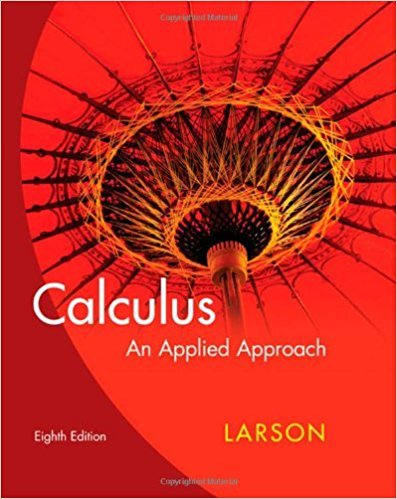×
×

# Solutions for Chapter 2.1: The Derivative and the Slope of a Graph## Full solutions for Calculus: An Applied Approach | 8th Edition

ISBN: 9780618958252Solutions for Chapter 2.1: The Derivative and the Slope of a Graph

Solutions for Chapter 2.1
4 5 0 295 Reviews
30
2
##### ISBN: 9780618958252

Chapter 2.1: The Derivative and the Slope of a Graph includes 75 full step-by-step solutions. Calculus: An Applied Approach was written by and is associated to the ISBN: 9780618958252. This textbook survival guide was created for the textbook: Calculus: An Applied Approach , edition: 8. This expansive textbook survival guide covers the following chapters and their solutions. Since 75 problems in chapter 2.1: The Derivative and the Slope of a Graph have been answered, more than 24029 students have viewed full step-by-step solutions from this chapter.

Key Calculus Terms and definitions covered in this textbook
• Conic section (or conic)

A curve obtained by intersecting a double-napped right circular cone with a plane

• Difference identity

An identity involving a trigonometric function of u - v

• Direct variation

See Power function.

• Distance (on a number line)

The distance between real numbers a and b, or |a - b|

• Divisor of a polynomial

See Division algorithm for polynomials.

• Domain of validity of an identity

The set of values of the variable for which both sides of the identity are defined

• Integrable over [a, b] Lba

ƒ1x2 dx exists.

• Inverse variation

See Power function.

• Line graph

A graph of data in which consecutive data points are connected by line segments

• Linear inequality in two variables x and y

An inequality that can be written in one of the following forms: y 6 mx + b, y … mx + b, y 7 mx + b, or y Ú mx + b with m Z 0

• Multiplication property of inequality

If u < v and c > 0, then uc < vc. If u < and c < 0, then uc > vc

• Natural logarithmic function

The inverse of the exponential function y = ex, denoted by y = ln x.

• Ordered set

A set is ordered if it is possible to compare any two elements and say that one element is “less than” or “greater than” the other.

• Period

See Periodic function.

• Product of matrices A and B

The matrix in which each entry is obtained by multiplying the entries of a row of A by the corresponding entries of a column of B and then adding

• Real number

Any number that can be written as a decimal.

• Scientific notation

A positive number written as c x 10m, where 1 ? c < 10 and m is an integer.

• Sum of two vectors

<u1, u2> + <v1, v2> = <u1 + v1, u2 + v2> <u1 + v1, u2 + v2, u3 + v3>

• Unit vector in the direction of a vector

A unit vector that has the same direction as the given vector.

• Zero matrix

A matrix consisting entirely of zeros.

×